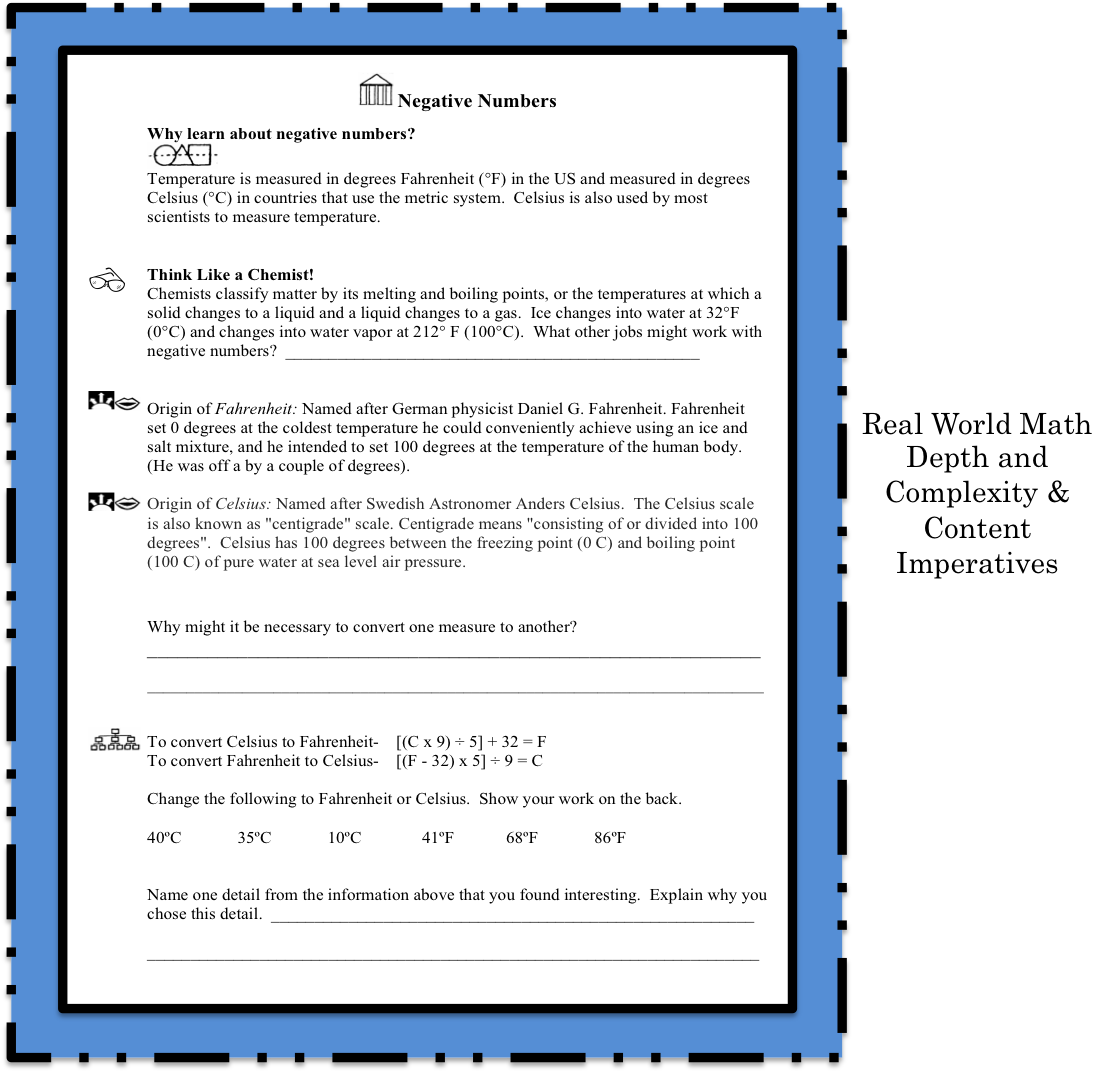# Depth and Complexity in Math

### How do the dimensions of depth and complexity fit into mathematics?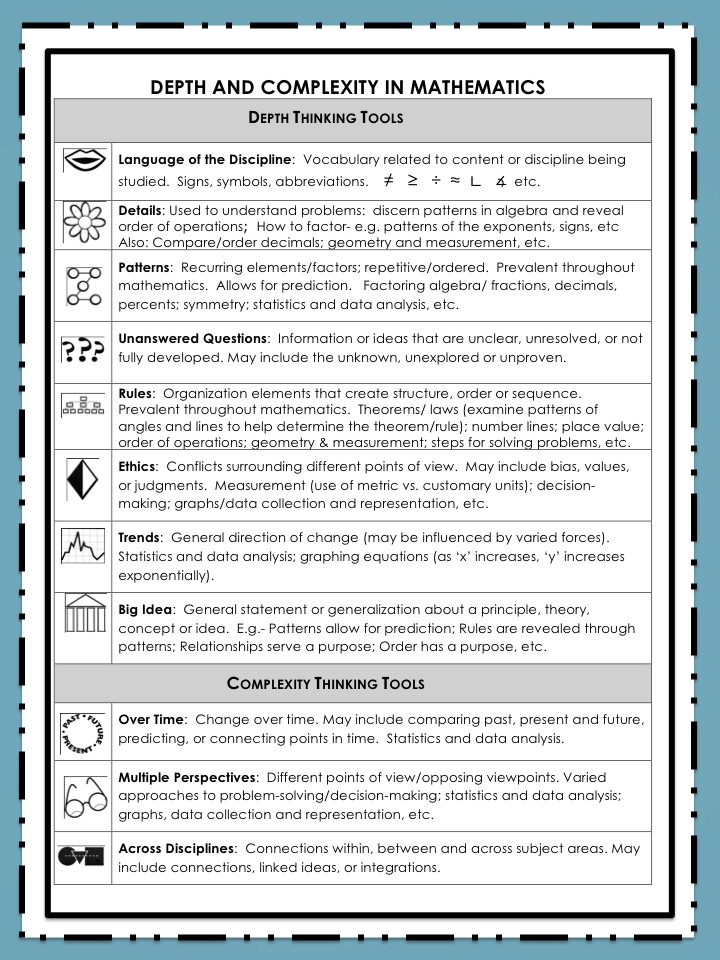Because the Depth and Complexity icons are tools that facilitate a more sophisticated understanding of a concept, they are not limited to a single content area.  These tools are a natural fit for developing a deeper understanding of mathematics.

Indeed, one cannot obtain mastery without being able to speak the language of math, or to understand the details of a number (or problem), or how a number (problem/or solution) is structured.

Consider the many ways these tools can be applied to math.

## Depth and Complexity in Math: Individual Icons

### Decimal Numbers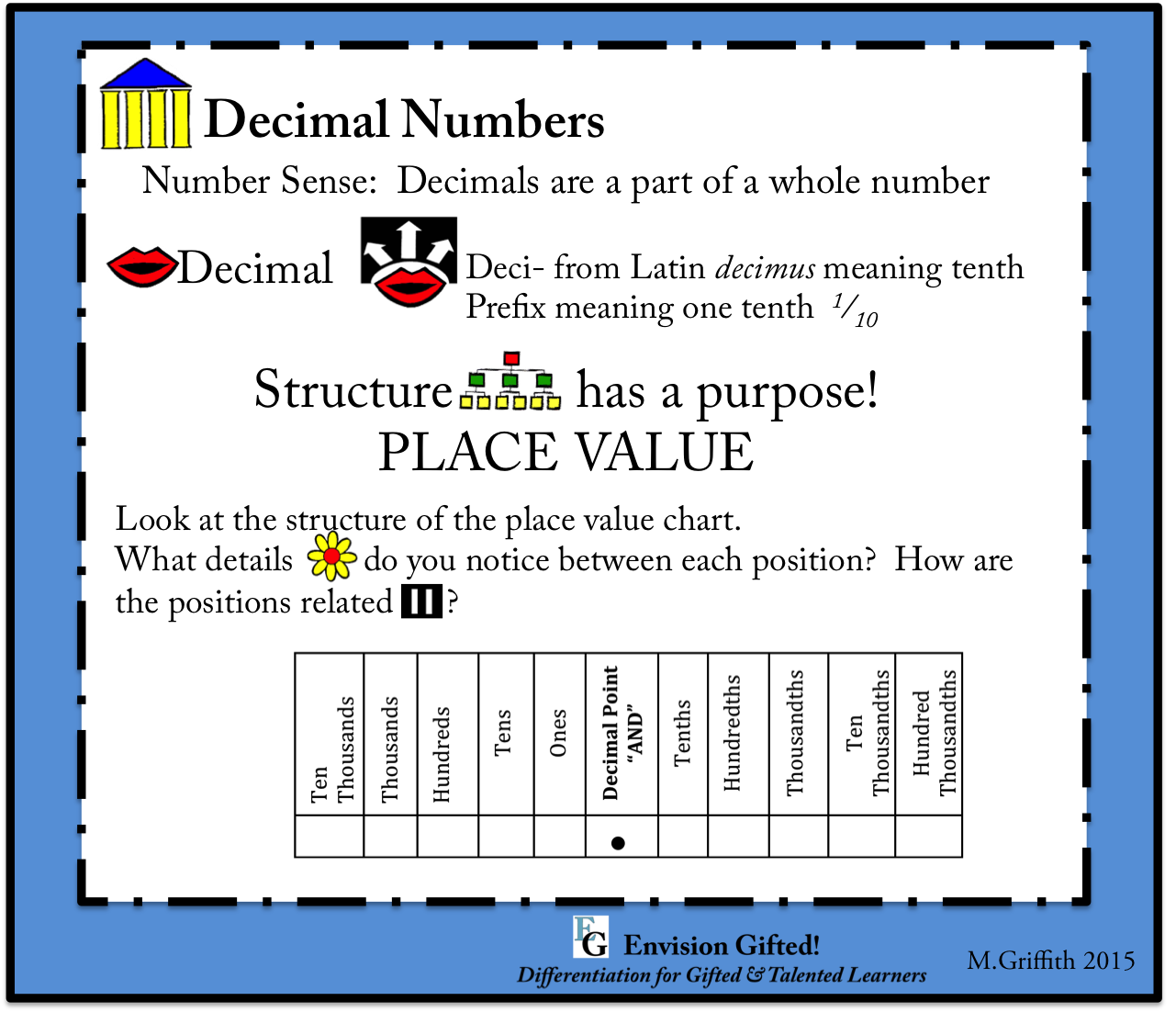Use the Depth and Complexity Framework to help students understand decimal numbers.  Often students rush to the operational manipulation of decimals without really understanding decimal numbers. This can leave them at a loss when asked to explain the concept, why they did what they did, or what their answer means.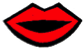Start by introducing students to the language of the discipline.  What is a decimal number?  Lead them to the understanding by discussing the origin of the word.Deci- from Latin  decimus meaning “tenth”.  You can also point out to students that “Deci” is a prefix that means one-tenth (1/10). This understanding ties nicely to the generalization, “STRUCTURE HAS A PURPOSE” and focusing on place value.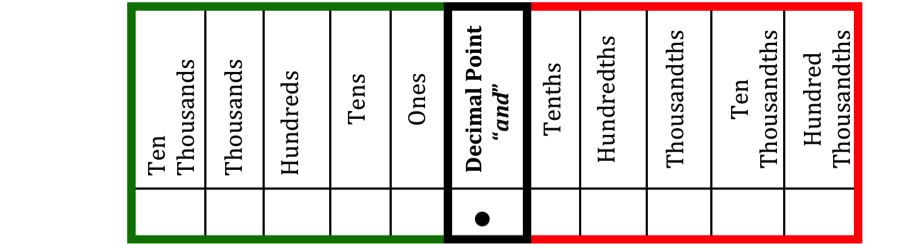Students working in partners or small groups- Have them examine a place value chart and look for details in the structure of the chart.  How are the place value positions related?

Direct students to write a multiplication rule to help get from the ones position to the tens position that would also hold true for the tens to the hundreds position, and the hundreds to thousands position, etc.

Ask students if that same rule holds true when applied to the positions to the right of the decimal point?  Why/why not?

### Differentiate Math: Compare and Order DecimalsStudents work with decimals to rank times from the Women’s Luge in the the 2014 Winter Olympics.  They then answer questions based on their findings.

File Compare Decimals Luge

## Frames of Knowledge- Depth and Complexity in Math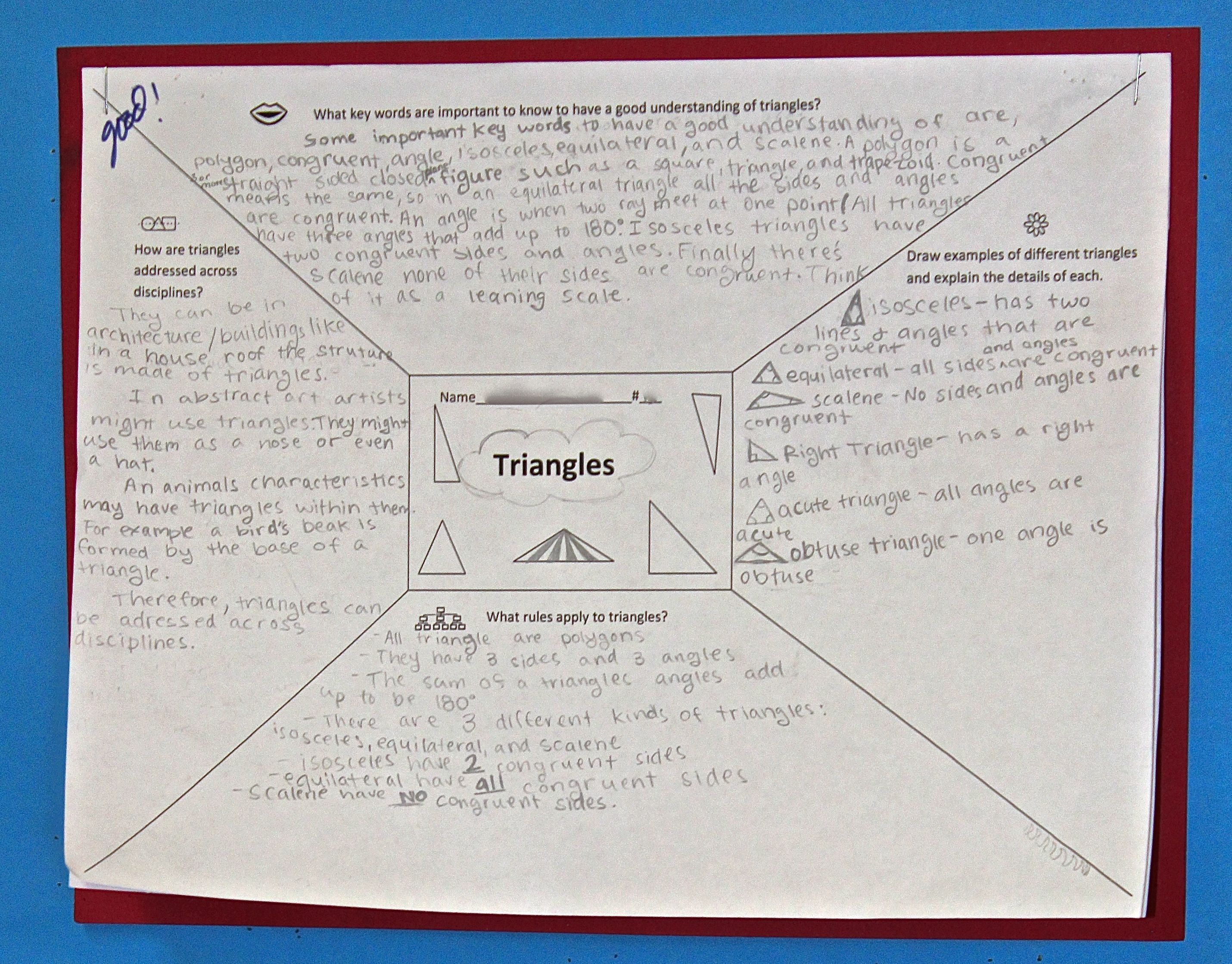Depth and Complexity Math: Triangles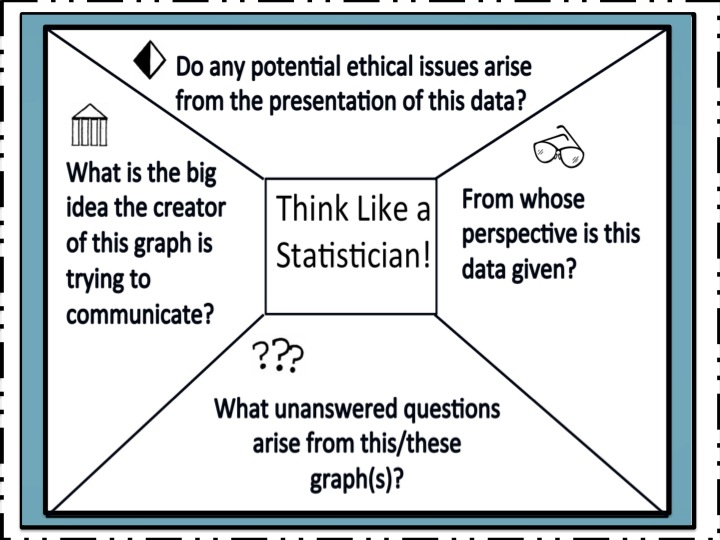TLAD Math Frame Depth and Complexity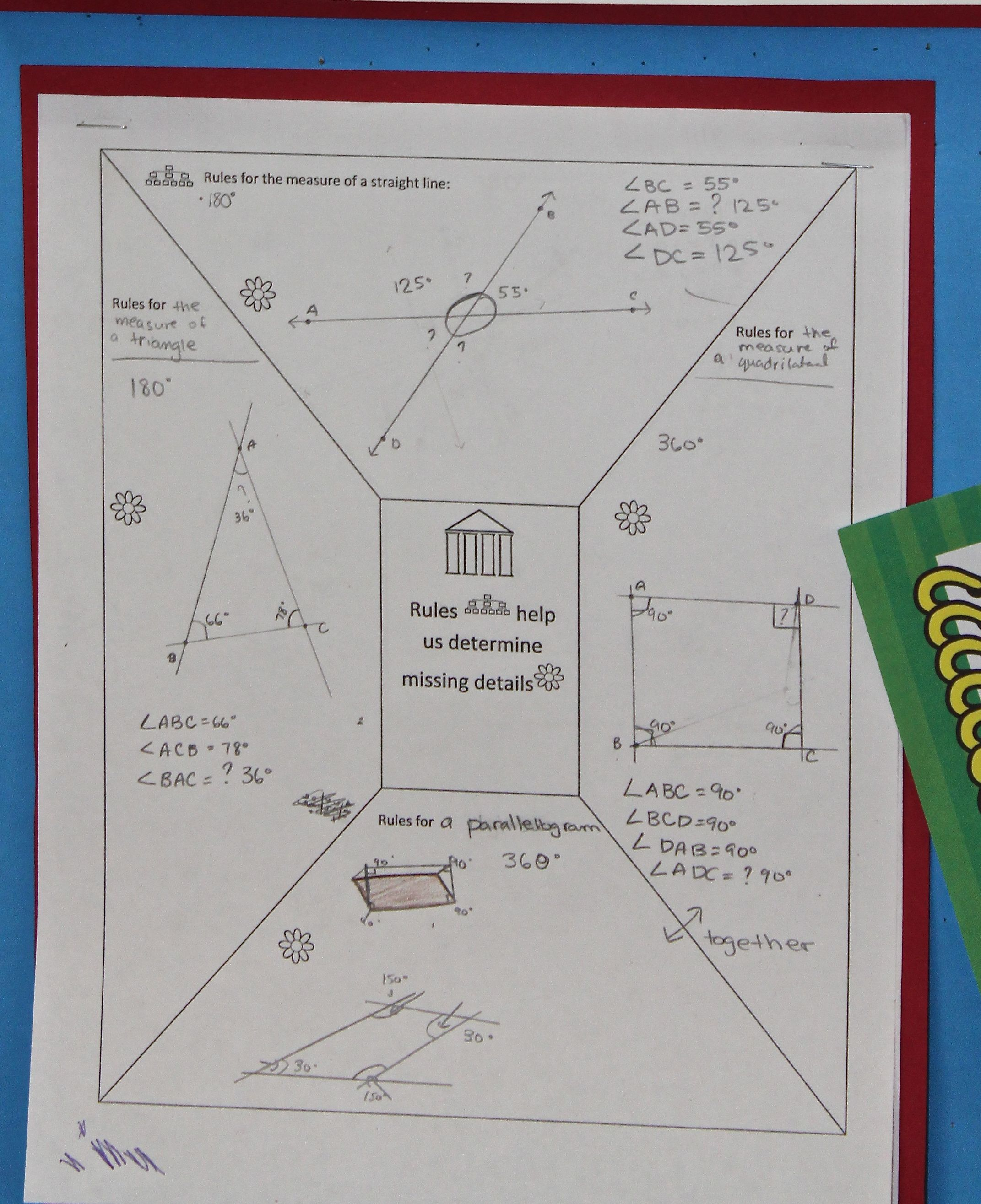Differentiate Math Frame: Depth and Complexity

## Independent Study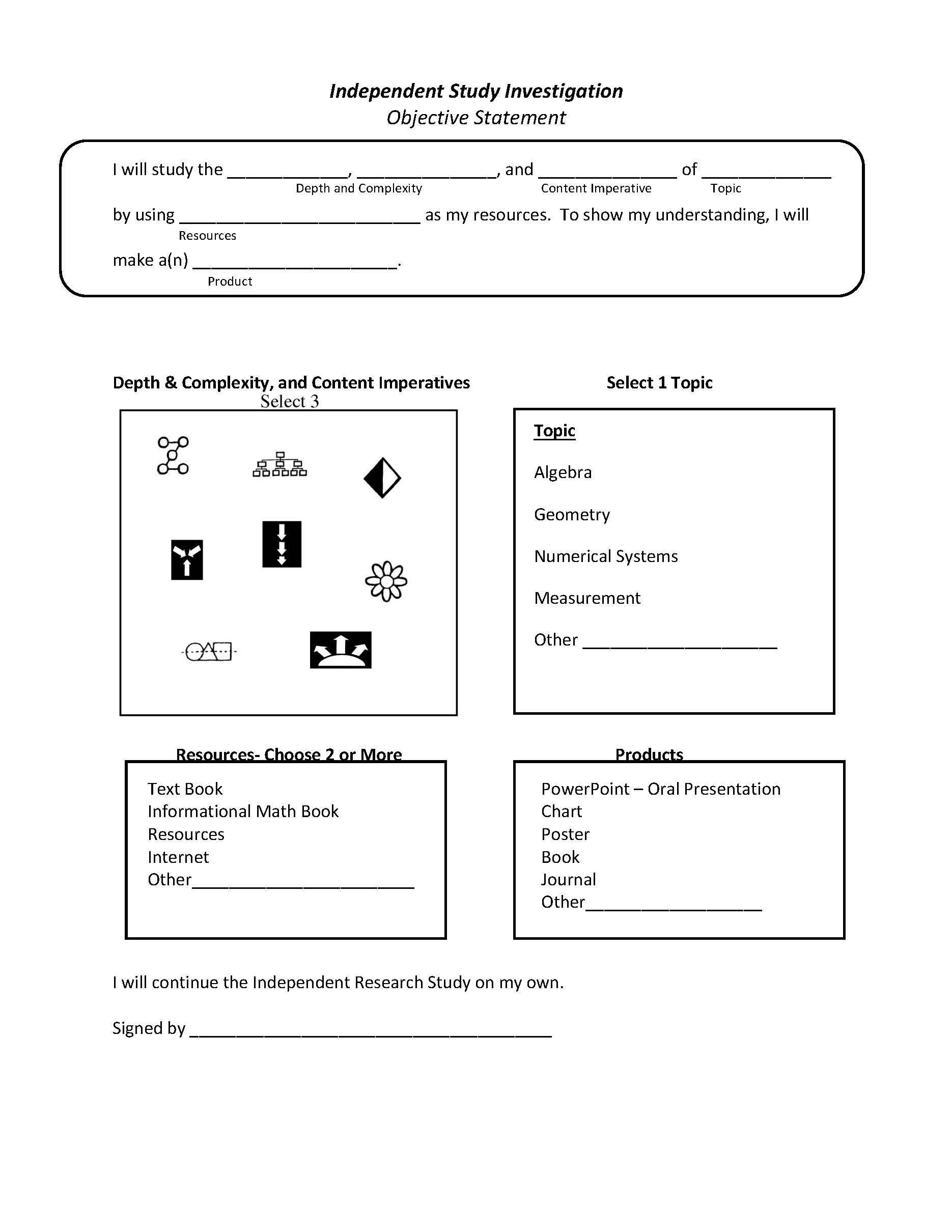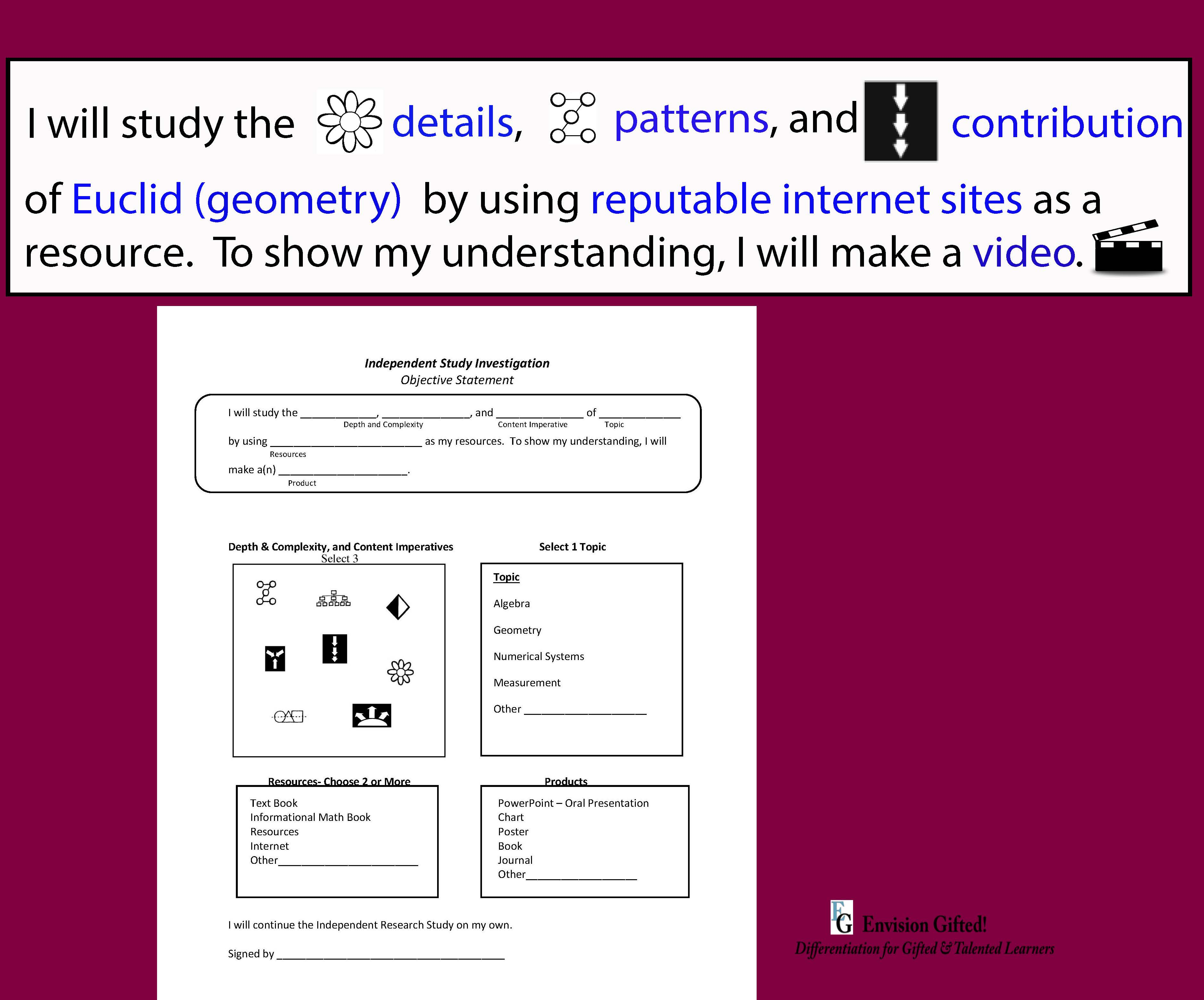If you have a student who has already demonstrated mastery of the content, consider having them complete an independent study.  Students (guided by the teacher) can use this form to create their own differentiated learning objective.   See example (right).

## Why learn about negative numbers?

Temperature is measured in degrees Fahrenheit (°F) in the US and measured in degrees Celsius (°C) in countries that use the metric system.  Celsius is also used by most scientists to measure temperature.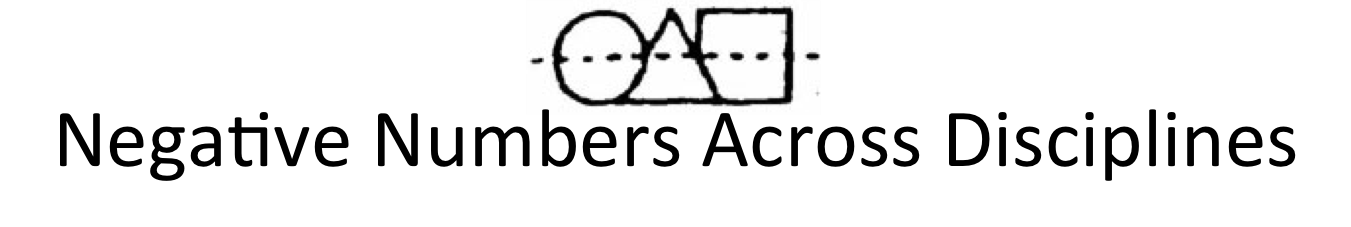Named after German physicist Daniel G. Fahrenheit. Fahrenheit set 0 degrees at the coldest temperature he could conveniently achieve using an ice and salt mixture, and he intended to set 100 degrees at the temperature of the human body. (He was off a by a couple of degrees).Origin of Celsius:

Named after Swedish Astronomer Anders Celsius.  The Celsius scale is also known as “centigrade” scale. Centigrade means “consisting of or divided into 100 degrees”.  Celsius has 100 degrees between the freezing point (0 C) and boiling point (100 C) of pure water at sea level air pressure.

Upload files for this lesson. Math Fahrenheit and Celsius. Envision Gifted. Depth and Complexity.

### Think Like a Chemist!

Chemists classify matter by its melting and boiling points, or the temperatures at which a solid changes to a liquid and a liquid changes to a gas.  Ice changes into water at 32°F (0°C) and changes into water vapor at 212° F (100°C).  What other jobs might work with negative numbers?

#### Why might it be necessary to convert one measure to another?